﻿ 并合双星系统的“共旋”引力波理论模型 A Theoretical Model of the “Co-Rotation” Gravitational Wave Theory of Two-Star System

Astronomy and Astrophysics
Vol. 06  No. 04 ( 2018 ), Article ID: 27372 , 14 pages
10.12677/AAS.2018.64007

A Theoretical Model of the “Co-Rotation” Gravitational Wave Theory of Two-Star System

Yueming Wu

Hangzhou Normal University, Hangzhou Zhejiang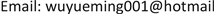Received: Oct. 10th, 2018; accepted: Oct. 24th, 2018; published: Oct. 31st, 2018ABSTRACT

The theoretical model of the “co-rotation” gravitational wave theory holds that the essence of gravity lies in “movement” and the material of the moving system has the function of “self-replication”. After the mathematical derivation and the application of Van der Pol equation, a formula for the unification of gravity and electromagnetic force was obtained. It is thought that gravitational waves are similar to DE Broglie material waves, and the moving objects are accompanied by one kind of fluctuation, and it is impossible to separate the movement of objects from the propagation of waves. It’s a quantitative calculation of the three predictions of Einstein’s mercury in motion, the bending of the light, the gravitational red shift. The calculated value corresponds to the measured value. It can also simulate the gravitational waves of pulsed binary stars, black hole binary stars and binary neutron star systems. The LOGO engineering experimental detection of gravitational wave data and “resonance” string theory calculation of two columns similar amplitude, spread in the opposite direction (relative to the system centre of mass) gravitational wave synthesis for amplitude modulation gravitational wave data better. It is a kind of theory of gravity that is worth exploring, which is of great significance to the understanding of the world.

Keywords:Theory of “Co-Rotation” Gravitational Waves, Self-Replication, Post Newtonian Approximation, Binary System, Gravitational Waves“共旋”引力波理论模型认为：引力本质在于“运动”，运动系统的物质均有“自我复制”功能。经过推导和运用范•德•波耳(Van der pol)方程求解，得到一个引力与电磁力相统一的公式。认为引力波类似德布罗意的物质波，运动着的物体都伴随着一种波动，而且不可能将物体的运动和波的传播分开。该式能对爱因斯坦的“水星进动、引力红移、光线偏折”三大预言进行定量计算，计算值与预测值相符。也能对脉冲双星、黑洞双星、双中子星系统引力波进行量化模拟。美国LIGO工程实验检测引力波数据与“共旋”弦理论计算的二列振幅相近、传播方向相反(相对系统质心)引力波合成为调幅引力波的数据有较好吻合。说明“共旋”弦理论是一值得探索的引力理论，该研究对认识世界有着深远意义。1. 引言

1916年，爱因斯坦在广义相对论中作了“水星进动、引力红移、光线偏折”三大预言。已经被许多物理学家设计出的观测实验所证实。笔者于2004年曾对《引力波与光线近日偏折》  进行定量计算。在“2005-国际物理年”提出“共旋”假说，出版了《共旋理论初探(上)——共旋引力波理论探索》专著，认为1997年由中科院王谦身、杨新社、汤克云等发现在日全食的“初亏”和“复圆”时二个“重力谷现象”是太阳和月球发出的二列引力波合成所引发 。2012年在“引力与相对论学会南充年会”上作了《对水星、脉冲星进动的比较研究》发言，由于“脉冲星是集自然界四大基本相互作用于一身的、极端物理条件下的‘天然实验室’” 。经过比较研究，2017年笔者将“共旋”假说提升为“共旋”弦理论 。研究证实自旋的星球均是引力波源，脉冲双星椭圆轨道的力心在双星的质心，也是在椭圆轨道的一个焦点上，认为并合双星系统椭圆轨道周期是双星(二列)引力波合成的调幅波周期。

“2016年2月11日，LSC (LIGO科学合作组织，LIGO Scientific Collaboration)向全世界宣布：人类首次直接探测到了引力波，并且首次观测到了双黑洞的碰撞与并合。”  “2017年10月16日，激光干涉引力波天文台(LIGO)科学合作组织和处女座引力波探测器(Virgo)合作组织联合召开发布会，宣布再次探测到时空的涟漪。这是人类第五次探测到引力波。”  我们认为：这是人类再次直接探测并证实爱因斯坦的“存在引力波”预言；但也证实引力波无需“时空弯曲”，当引力波的频率在声波波段时人类能听到引力波。“共旋”引力波理论模型能对并合双星系统的引力波进行定量研究，期盼专家学者斧正。

2. “共旋”弦理论认为“引力波是简谐波”

2007年笔者参加了中国地球物理学会天灾预测专业委员会，在天灾预测实践过程中，认识到地球人应加强对地球电磁场的研究。2012年笔者运用物理方法统一了引力场和电磁场，得到一个描述引力、电

$F=W\left\{\mathrm{cos}\left[\psi \right]-Y\mathrm{sin}\left[\psi \right]\right\}$ (1)

$F=\frac{GMm}{{r}^{2}}\mathrm{cos}\left[\psi \right]±\frac{KMm}{{r}^{2}}Y\mathrm{sin}\left[\psi \right]$ (2)

(2)式是炎黄子孙提出的“共旋”弦理论的力公式。是一个描述引力及电力本质及其产生机制(包括质量、惯性)的宇宙公式，也是可描述四种基本力(引力、弱力、电磁力和强力)相互作用规律的统一理论公式。公式非常简洁和壮丽。杨振宁在他的《美与物理学》中谈到对狄拉克的文章感受时说：“数学的最高境界是结构美，是简洁的逻辑美，因此他的文章也就是给读者‘秋水文章不染尘’”的感受。杨先生在对牛顿、麦克斯韦、爱因斯坦、狄拉克、海森堡等人的方程式评价时说：“它们以极度浓缩的数学语言写出了物理世界的基本结构，可以说是造物者的诗篇。” 

“共旋”弦理论公式(2)表明星球引力波是一星一波源，波频率与星球的自转频率一致。认为自转的星球均能发出引力波，导电体结构星球还会发出电磁波。因为导电物质构成的自旋星球会“共旋”起电，形成如中华文化中太极图式的电偶极子。地球的自转运动使铁、镍组成的地核成为电偶极子，由于铁、镍是金属导体，不像太阳是由等离子气体组成的星球，不同电荷间有很强恢复力而互为屏蔽；铁、镍导体间的不同电荷会湮灭(短路)使外核熔融为液态，使地球成为电球和磁球。自转的地球既发出引力波，从地球的地核核面也会发出电磁波(地球表面的磁场就是由地核面发出电磁波引起的)。太阳系中的星球自转

3. 水星近日点进动的计算 

${h}^{2}{u}^{2}\left(\frac{{\text{d}}^{2}u}{\text{d}{t}^{2}}+u\right)=-\frac{F}{m}$ (3)

$\text{d}\theta =\frac{h\cdot \text{d}r}{r\sqrt{\frac{2E\text{}}{m}{r}^{2}+2{k}^{2}r-{h}^{2}}}$ (4)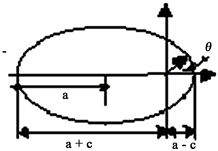Figure 1. Schematic diagram of mercury’s orbit around the sun

$\text{d}\theta =\frac{h\cdot \text{d}r}{r\sqrt{-\frac{GM\text{}}{a}{r}^{2}+2GM\cdot r-{h}^{2}}}$ (5)

$\text{d}\theta =\frac{h\cdot \text{d}r}{r\sqrt{GM\left(s\cdot {r}^{4}/2\cdot a-s{r}^{3}-{r}^{2}/a+2\cdot r\right)-{h}^{2}}}$ (6)

$G=6.672×{10}^{-11}{\text{m}}^{\text{3}}/\text{kg}\cdot {\text{s}}^{\text{2}}$$M=1.989×{10}^{30}\text{\hspace{0.17em}}\text{kg}$$a=5.786×{10}^{10}\text{\hspace{0.17em}}\text{m}$$e=0.2056$$p=\left(1-{e}^{2}\right)\cdot a$$h=\sqrt{p\cdot GM}$${c}_{1}=2.998×{10}^{8}\text{m}/\text{s}$$\omega =\frac{2\text{π}}{27.28×24×3600}$

$s=\frac{{\omega }^{2}}{{c}_{1}^{2}}=7.89589×{10}^{-29}\left(1/{\text{m}}^{\text{2}}\right)$

$\theta 0={\int }_{a-c\text{}}^{a+c}\frac{h\cdot \text{d}r}{r\sqrt{-G\cdot M\cdot {r}^{2}/a+2\cdot G\cdot M\cdot r-{h}^{2}}}=3.141592602285\left( rad \right)$

$\theta 1={\int }_{a-c\text{}}^{a+c}\frac{h\cdot \text{d}r}{-r\sqrt{GM\left(s\cdot {r}^{4}/2a-s{r}^{3}-{r}^{2}/a+2\cdot r\right)-{h}^{2}}}=3.141592855262915\left( rad \right)$

$\text{d}\theta =\theta 1-\theta 0=2.52621×{10}^{-7}\left(\text{rad}\right)$

4. 脉冲星是集自然界四大基本相互作用于一身的“天然实验室”

“1974年，Hulse和Taylor发现了第一对脉冲双星PSR1913+16，并对它的轨道运动进行了长期观测。该系统中，一颗59毫秒的射电脉冲星，以最大可达3%光速的速度在一个椭率为0.6的椭圆轨道上围绕另外一颗中子星以7.75小时的轨道周期旋转。……最新的数据 表明(Weisberg 2004)这对双星的质量分别为1.4414 +/− 0.0002和1.38674 +/− 0.0002倍的太阳质量，椭圆轨道近星点的进动为每年4.226595 +/− 0.0009度(建立广义相对论时起重要作用的水星近星点的反常进动值仅为每100年43角秒)。” 

“共旋”引力波理论认为：每颗脉冲星都是引力波(电磁波)的波源，脉冲双星PSR1913+16的公共质心在它们的椭圆轨道的一个焦点上。相对沿各自的椭圆轨道运行。见图2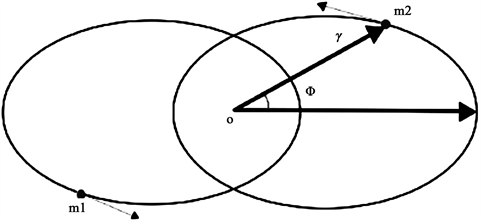Figure 2. Schematic diagram of PSR1913+16 operating orbit of pulse binary star

$M=m1+m2=5.62887×{10}^{30}\text{\hspace{0.17em}}\text{kg}$ ；脉冲双星的轨道周期为； $T=27906.98161\text{\hspace{0.17em}}\text{s}$ ；轨道偏心率： $e=0.617138$ ，于1975年观测双星之一的自转周期P(秒)为：0.059030，(于1993年减慢至：0.0590299952695土8；)。

${Q}_{m1}=±\lambda \frac{m1\cdot {\omega }_{m1}\cdot {R}_{m1}}{{c}_{1}}=8.42859×{10}^{17}\text{\hspace{0.17em}}\text{C}$

$a1=\sqrt{\frac{\lambda \cdot M\cdot {T}^{2}}{4{\text{π}}^{2}}}=2.12264×{10}^{9}\text{\hspace{0.17em}}\text{m}$$c1=a1\cdot e=1.30996×{10}^{9}\text{\hspace{0.17em}}\text{m}$

${\theta }_{0mk}={\int }_{a1-c1\text{}}^{a1+c1}\frac{h1\cdot \text{d}r}{r\sqrt{-\lambda \cdot M\cdot {r}^{2}/a1+2\cdot \lambda \cdot M\cdot r-h{1}^{2}}}=3.14159-1.37693×{10}^{-8}i$

$F1\text{}=\lambda \cdot m1\cdot \mathrm{cos}\left[{\omega }_{m1}\cdot t+\phi 1\right]=\lambda \cdot M\cdot \mathrm{cos}\left[{\omega }_{m1}\cdot t\right]=4.84479×{10}^{20}\mathrm{cos}\left[106.441\cdot t\right]$

$F2=\lambda \cdot m2\cdot \mathrm{cos}\left[{\omega }_{m2}\cdot t+\phi 2\right]=\lambda \cdot M\cdot \mathrm{cos}\left[{\omega }_{m2}\cdot t\right]=4.84479×{10}^{20}\mathrm{cos}\left[106.442\cdot t\right]$ ；则合成振动为： $F=F1+F2=2\lambda \cdot M\cdot \mathrm{cos}\left[\frac{\Delta \omega }{2}t\right]\cdot \mathrm{cos}\left[\frac{{\omega }_{m2}+{\omega }_{m1}}{2}t\right]$ ；其中： $\Delta \omega ={\omega }_{m2}-{\omega }_{m1}$ ；考量到 ${\omega }_{m1},{\omega }_{m2}\gg \Delta \omega$ ，故上式表明合成振动是一个振幅作低频变化的高频振动，通常称此处的 $\mathrm{cos}\left[\Delta \omega /2\right]$ 为低频包络因子，或低频调幅因子。从图3可见，波包重复出现的周期 ${T}_{21}$$\mathrm{cos}\left[\Delta \omega t/2\right]$ 函数周期，即拍频是此函数频率的二倍， ${\omega }_{21}=2×\frac{\Delta \cdot \omega }{2}=\Delta \cdot \omega$ ，……这表明，嗡嗡嗡声的拍频就等于差频。  ；作 $plot\left[F,\left\{t,0,T\right\}\right]$ 得到如图3、的拍频曲线。

$\text{d}\theta =\frac{h\cdot \text{d}r}{r\sqrt{\lambda \cdot M\left(s\cdot {r}^{4}/2\cdot a-s{r}^{3}-{r}^{2}/a+2\cdot r\right)-{h}^{2}}}$ (9)

${\theta }_{1mk}={\int }_{a1-c1}^{a1+c1}\frac{h1\cdot \text{d}r}{r\text{}\sqrt{-\lambda \cdot M\cdot \left(\frac{s21}{2a1}{r}^{4}-s21\cdot {r}^{3}-\frac{{r}^{2}}{a1}+2\cdot r\right)-h{1}^{2}}}=3.14163-2.11608i$

$\text{d}\theta k={\theta }_{1mk}-{\theta }_{0mk}=0.0000326311-2.11608i$ ；一年时间里的进动值为：

$\text{d}\theta k×2×57.2957795×\frac{365.25×24×3600}{T}=4.228\left(度/年\right)$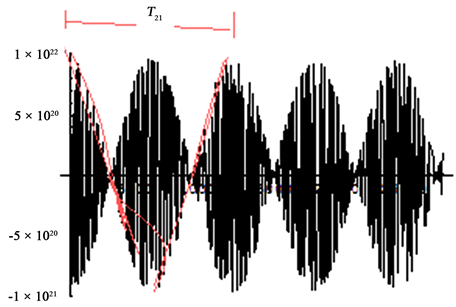Figure 3. Beat frequency curve of the time synthesis of the two columns of pulse-binary PSR1913+16 gravitational waves

5. 并合双星系统的“共旋”引力波理论模型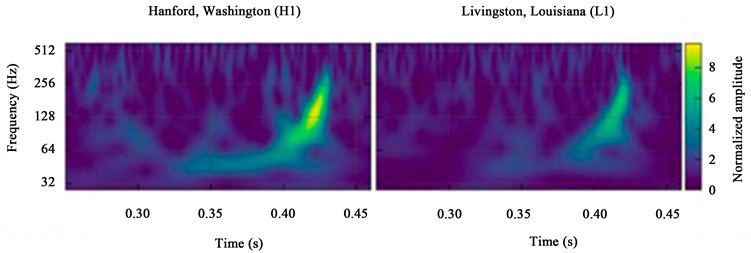Figure 4. The measured gravitational wave data of the collision and merger of the twin black holes in LIGO project 20150914 in the United States. Left: LIGO Hanford, right: Livingston

5.1. 双黑洞系统是一个独立的、发出调幅引力波、最后走向并合的双星系统

${R}_{hd1bc}=8.25×{10}^{4}$${R}_{hd2bc}=7.676×{10}^{4}$ 分析图5中的黑洞2、初始位置从近双黑洞系统质心位置开始轨道运行，双星系统演化过程的旋进早期，双星间距较大，根据 LIGO工程20150914双黑洞碰撞与并合的实测引力波数据，将双黑洞运行初始轨道运行周期定为每秒31圈： $T0=1/31$ ，计算出初始轨道角速度为： $\omega 0=2\text{π}/T0=194.787\left(\text{rad}/\text{s}\right)$ ；在牛顿引力理论框架下，根据开普勒第三定律，计算黑洞1和黑洞2的椭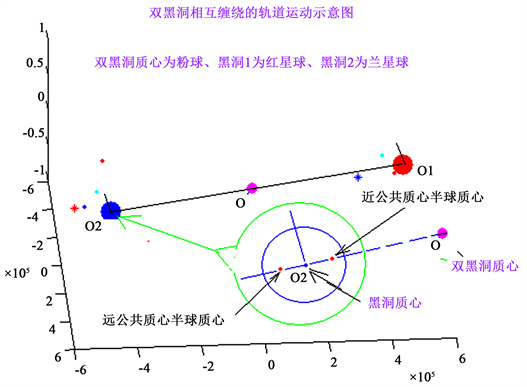Figure 5. Schematic diagram of angular momentum transfer process of dual black hole system

“距离是一千公里，”左右的实际，取轨道偏心率： $e1=0.08$ 则：黑洞1和黑洞2的椭圆轨道半短轴分别为 $c10=a10\cdot e1=40101\left(\text{m}\right)$$c20=a20\cdot e1=37313\left(\text{m}\right)$ ；计算黑洞2在初始近双黑洞质心的距离为： $rhd20j=a20-c20=4.29×{10}^{5}\left({\text{m}}^{\text{2}}\right)$ ；则近公共质心半球绕公共质心O自西向东运动的线速度为： $vhd20j=w0×\left(rhd20j-Rhd2bc\right)=6.8627×{10}^{7}\left(\text{m}/\text{s}\right)$ ；远公共质心半球绕公共质心O自西向东运动的线速度为： $vhd20y=9.853×{10}^{7}\left(\text{m}/\text{s}\right)$ ；显然黑洞2远双黑洞质心半球的角动量比近半球大得多，相对黑洞2的质心而言，黑洞2远双洞质心半球的角动量为

$Lhd20y=\left(m2/2\right)×vhd20y×Rhd2bc=2.1824×{10}^{44}\left(\text{kg}\cdot {\text{m}}^{\text{2}}/\text{s}\right)$

5.2. 共旋引力波理论“后牛顿近似方法”能模拟双黑洞系统旋进、并合和铃振3个阶段Table 1. Data calculation table of the three stages of the elliptical orbit collision and merger of double black holes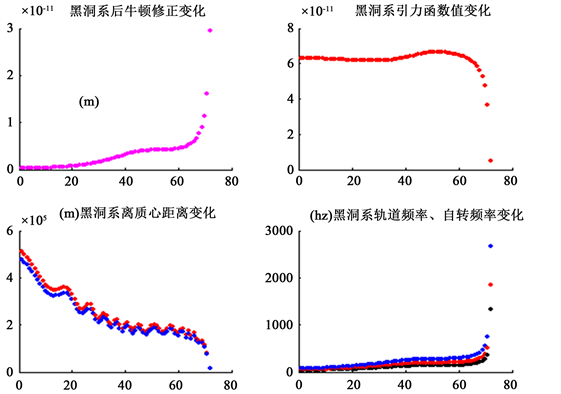Figure 6. MATLAB programs calculate some dynamic changes of physical values during the twin-black hole entangled orbit. (upper left) variation of Newton correction coefficient after double black hole system; (upper right) the dynamic change of the gravitational function after the double black hole system; (lower left) the dynamic change of the distance from the center of mass of the double black hole system; (lower right) dynamic changes of the orbital motion and rotation frequency of the double black hole system

6. “共旋”引力波理论模型对中子星引力波及其电磁对应体的探索

“2017年北京时间10月16日22点，激光干涉引力波天文台(LIGO)科学合作组织和处女座引力波探测器(Virgo)合作组织联合召开发布会，宣布再次探测到时空的涟漪。这是人类第五次探测到引力波。然而科学界的兴奋之情甚至不亚于第一次探测到引力波时。因为与之前被探测到的四个引力波信号不同，这次探测到的引力波信号GW170817来自1.3亿光年外两颗并合的中子星，而且科学家第一次同时观测到了引力波及其电磁对应体，以及科学家预言的巨新星现象。” 

7. 结论与讨论

1) 共旋引力波理论认为：引力不是物体本身固有的，也不是“时空弯曲”，而是物体自转运动的结果。一个自旋的系统，系统中每个质点均会产生向心力，该力作为受迫振动的强迫力使系统共振，稳定的共振状态的自旋系统会从自转轴中心始发出频率与自旋频率相同，位移振幅为： $A=GM$ 的引力波。引力波为简谐波。物体之间相互作用力的传递是通过引力波实现相互作用的。各星球发出的引力波是不同的。不同自转角速度的星球发出不同频率的引力波。

2) $F=\frac{GMm}{{r}^{2}}\cdot \left(1-\frac{1}{2}s\cdot {r}^{2}\right)$ 式是“共旋”理论的“后牛顿力”表达公式。该式能对爱因斯坦的“水星

3) 共旋引力波理论能够解释美国LIGO工程探测到双黑洞、双中子星的碰撞与并合的全过程。说明东方的共旋引力波理论得到西方的GW150914实验引力波实测数据的验证。说明共旋引力波理论认为的：“一星一波源，双黑洞在相互旋绕的过程中，从力心发出二列引力波频率相近、传播方向相反，会使二列引力波的迭加合成调幅引力波”得到实验的支持。该引力波的频率又在可闻声波频段之内，说明人类也能听到引力波；双中子星的碰撞与并合过程中自转速度接近或达到光速时，还会发出伽马射线暴等电磁信号。

4) 美国LIGO工程探测到双黑洞、双中子星的碰撞与并合的全过程。证明人类再次证实爱因斯坦“存在引力波”的预言，同时也证明：时空不会弯曲，时空无需弯曲。证实我们在2005年创建的共旋引力波基础理论  ，得到了“LIGO官网11日在一份新闻稿中表示，此次探测到的引力波是由两个黑洞合并引发的。”实测的支持。

A Theoretical Model of the “Co-Rotation” Gravitational Wave Theory of Two-Star System[J]. 天文与天体物理, 2018, 06(04): 88-101. https://doi.org/10.12677/AAS.2018.64007

1. 1. 伍岳明. 引力波与光线近日偏折[J]. 浙江工业大学学报, 2004(1): 99-104.

2. 2. 伍岳明, 曹明富. 共旋理论初探上册——共旋引力波理论探索[M]. 科学技术文献出版社, 2005: 7, 40, 43-47, 79-80.

3. 3. 李柯伽, 乔国俊, 徐仁新, 张承民, 朱宗宏, 王洪光. 脉冲星和引力波[C]//第7届亚太引力与天体物理国际会议会议文集. 2005: 10-17.

4. 4. 伍岳明. 炎黄子孙的“共旋”弦理论与土星光环的形成机制[J]. 天文和天体物理, 2017, 5(4): 29-44.

5. 5. 陈雁北, 范锡龙. 爱因斯坦都不敢想象, 我们探测到引力波[EB/OL]. 凤凰资讯. 中国青年网. 2016-02-13.

6. 6. 陈雁北, 范锡龙. 最强引力波信号出现 一图读懂它和你什么关系[EB/OL]. 凤凰资讯. 中国青年网. 2016-02-13.

7. 7. 倪简白, 主编. 物理新论[M]. 长春: 长春出版社, 2013: 1-15.

8. 8. 周衍柏. 理论力学教程(第二版) [M]. 北京: 高等教育出版社, 1986: 65-83.

9. 9. 李宗伟. 脉冲双星PSR1913+16——1993年诺贝尔物理学奖[J]. 大学物理, 1994(3): 1-6.

10. 10. 程守洙, 江之永. 普通物理学(第四版) [M]. 北京: 高等教育出版社, 1982: 172-176.

11. 11. 钟锡华, 周岳明. 力学[M]. 北京: 北京大学出版社. 2000, 12(1): 178-193, 228-230.

12. 12. 伍岳明, 曹明富. 形成日月岁差机制的定量研究[J]. 地球科学前沿, 2015, 5(5): 371-378.